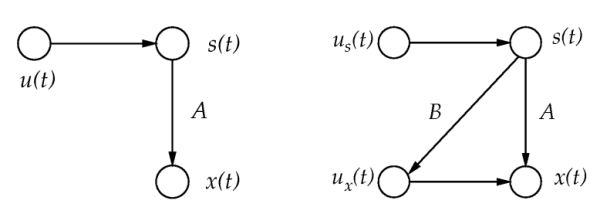# Hierarchical modeling of variances

In many models, variances are assumed to have constant values although this assumption is often unrealistic in practice. Joint modeling of means and variances is difficult in many learning approaches, because it can give rise to infinite probability densities. In Bayesian MCMC methods where sampling is employed, the difficulties with infinite probability densities are avoided, but these methods are not efficient enough for very large models. Our variational Bayesian method, which is based on the building blocks framework, is able to jointly model both variances and means efficiently (Valpola et al., 2004).

The basic building block in our variance models is the variance node, which is a time-dependent Gaussian variable controlling the variance of another time-dependent Gaussian variable. The variance node can be used together with our other Bayes building blocks to construct various hierarchical models for the variances. Such models are introduced and discussed in (Valpola et al., 2004). In this paper, we have applied them to biomedical MEG data consisting of magnetic measurements of human brain signals contaminated by various artefacts. The experiments in (Valpola et al., 2004) clearly demonstrate the existence of variance sources in such a data.The above figure shows two examples of models where variance nodes are employed. On the left, the variance node generates a supergaussian density to the source and hence enables the model to perform source separation. On the right, the noise process of the observations is also conditioned on the sources.

### References

H. Valpola, M. Harva, and J. Karhunen, "Hierarchical models of variance sources". Signal Processing vol. 84, no. 2, 2004, pp. 267-282. Pdf (1128k)

You are at: CISResearchAlgorithmsBayes → Research

Page maintained by juha.karhunen at hut.fi, last updated Wednesday, 01-Oct-2008 10:29:01 EEST